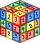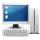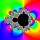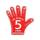# Tenth member

Calculate the tenth member of geometric sequence when given: a1=1/2 and q=2

Result

x =  128

#### Solution:Leave us a comment of example and its solution (i.e. if it is still somewhat unclear...):

Showing 0 comments:Be the first to comment!## Next similar examples:

1. SequenceFind the common ratio of the sequence -3, -1.5, -0.75, -0.375, -0.1875. Ratio write as decimal number rounded to tenth.
2. GP membersThe geometric sequence has 10 members. The last two members are 2 and -1. Which member is -1/16?
3. Geometric sequence 4It is given geometric sequence a3 = 7 and a12 = 3. Calculate s23 (= sum of the first 23 members of the sequence).
4. QuotientDetermine the quotient and the second member of the geometric progression where a3=10, a1+a2=-1,6 a1-a2=2,4.
5. Geometric progression 2There is geometric sequence with a1=5.7 and quotient q=-2.5. Calculate a17.
6. Five membersWrite first 5 members geometric sequence and determine whether it is increasing or decreasing: a1 = 3 q = -2
7. GP - 8 itemsDetermine the first eight members of a geometric progression if a9=512, q=2
8. SequenceBetween numbers 1 and 53 insert n members of the arithmetic sequence that its sum is 702.
9. Six termsFind the first six terms of the sequence a1 = -3, an = 2 * an-1
10. Geometric progression 48,4√2,4,2√2
11. ComputerThe computer was purchased 10000,-. Each year, the price of a computer depreciates always the same percentage of the previous year. After four years, the value of the computer is reduced to 1300,- How many percent was depreciated price of the computer each
12. Geometric progressionFill 4 numbers between 4 and -12500 to form geometric progression.
13. A perineumA perineum string is 10% shorter than its original string. The first string is 24, what is the 9th string or term?
14. ValueFind the value of the expression: 6!·10^-3
15. EquationHow many real roots has equation ? ?
16. Theorem proveWe want to prove the sentense: If the natural number n is divisible by six, then n is divisible by three. From what assumption we started?
17. EquationEquation ? has one root x1 = 8. Determine the coefficient b and the second root x2.